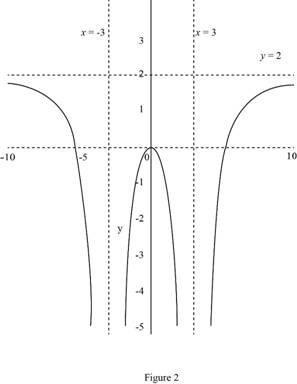# The graph of f ( x ) which satisfies the conditions, lim x → 3 f ( x ) = − ∞ , lim x → ∞ f ( x ) = 2 , f ( 0 ) = 0 , f is even.### Single Variable Calculus: Concepts...

4th Edition
James Stewart
Publisher: Cengage Learning
ISBN: 9781337687805### Single Variable Calculus: Concepts...

4th Edition
James Stewart
Publisher: Cengage Learning
ISBN: 9781337687805

#### Solutions

Chapter 2.5, Problem 10E
To determine

## To sketch: The graph of f(x) which satisfies the conditions, limx→3f(x)=−∞,limx→∞f(x)=2,f(0)=0, f is even.

Expert Solution

### Explanation of Solution

Recall the definition of horizontal asymptote, “The line y=L is called a horizontal asymptote of the curve y=f(x) if limxf(x)=L or limxf(x)=L”.

Recall the definition that the line x=a is said to be a vertical asymptote of the function f(x), if at least one of the following conditions must be true:limxaf(x)=±, limxa+f(x)=±, limxaf(x)= and limxaf(x)=.

The limx3f(x)= means that means that x=3 is a vertical asymptote to the function f. That is, as x approaches 3, the graph of the curve approaches negative infinity.

The limxf(x)=2 means that y=2 is a horizontal asymptote to the function f as x approaches infinity.

The graph must contain a point (0, 0) as f(0)=0. So, mark the point (0, 0) as a filled dot.

Here, the function f is even. That is, f(x)=f(x).

Since limx3f(x)=, limxf(x)=2 and f is even, write limx3f(x)= and limxf(x)=2.

Thus, the possible graph of f(x) is shown below in Figure 1.

Graph:

Use the above information and obtain the graph of f as shown below in Figure 1.Thus, the required graph of f.

### Have a homework question?

Subscribe to bartleby learn! Ask subject matter experts 30 homework questions each month. Plus, you’ll have access to millions of step-by-step textbook answers!